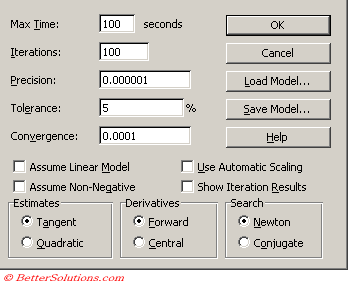### Solver OptionsMax Time - Sets the maximum amount of time that Solver will spend to try and find a valid solution.
Iterations - Defines the limit of the number od attempts that Solver should use to try and find a satisfactory solution.
Precision - This pertains to the constraints. The precision given here refers to the precision with with the constraints have to be met.
Tolerance - This pertains to integer constraints. You should always try and find a solution without an integer constraint ?
Convergence - Defines the amount of relative change to allow in the last five iterations before Solver stops with a solution.
Assume Liner Model - This defines which algorithm to use to try and solve the model. Linear models are easier to solve.
Assume Non-Negative - This ensures that all the decision variables which do not have a constraint attached to them have a lower bound of zero.
Use Automatic Scaling -
Show Iteration Results -

Tangent - Select when the model is linear.
Quadratic - Select when the model is non linear.
Forward - Selecting this option will potentially speed up Solver when the iterations are very slow due to the constraints.
Central - This can be used to improve the accuracy when the cells which have constraints change by a large amount.
Newton - This uses more memory but requires fewer iterations to obtain the solution.
Conjugate - This uses less memory and should be used for more complicated and larger models. You may alos need to increase the number of iterations.